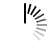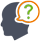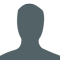Diwali Bonanza | Get 30-Days Free Fun-Filled Learning | Subscribe Now1800-833-6464 Buy Activation Why Tutorix Features Login

#DoubtTushar

In figure below, if $AB\ ⊥\ BC$, $DC\ ⊥\ BC$, and $DE\ ⊥\ AC$, prove that $Δ\ CED\ ∼\ Δ\ ABC$.Solutions :Akhilesh

Given:

In the given figure $AB\ âŠ¥\ BC$, $DC\ âŠ¥\ BC$, and $DE\ âŠ¥\ AC$.

To do:

We have to prove that $Î”\ CED\ ∠¼\ Î”\ ABC$.

Solution:

In $\vartriangle ABC$ and $\vartriangle CED$,

$\angle BAC+\angle BCA=90^o$

$\angle BCA+\angle ECD=90^o$

This implies,

$\angle BAC=\angle ECD$

In $\vartriangle ABC$ and $\vartriangle CED$,

$\angle ABC=\angle CED=90^o$

$\angle BAC=\angle ECD$

Therefore,

$Î”\ CED\ ∠¼\ Î”\ ABC$.

Hence proved.

1

﻿